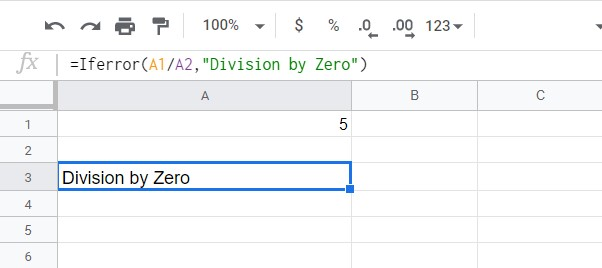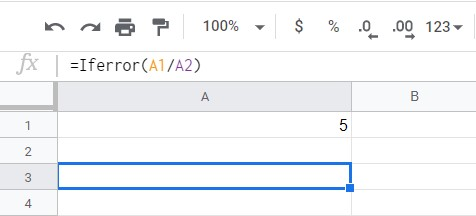# How to Get Rid of #Div/0 in Google Sheets

Using automatic formulas in Google Sheets is more of a necessity than a choice when dealing with a large amount of data. Automation, however, can come with a few downsides, such as errors resulting from improper mathematical processes. Dividing by zero, or the #Div/0 error, is one of these.In this article, we’ll show you how to get rid of the #Div/0 error in Google Sheets.

## Populate the Cells Properly

As mentioned above, you get a #Div/0 error if you divide anything by zero. It’s an equation that results in a mathematical impossibility and thus isn’t accepted by the program. This error can be avoided simply by making sure that no formula uses zero or a blank cell as a divisor. You can either delete or populate blank cells, or not include them in the equation at all. This method is fine if you’re managing a small number of cells, but for large automated formulas, you’ll need a catch-all code.

## Using the If Error Function

If you’re using a formula to automatically calculate the values of cells, errors like #Div/0 are to be expected. What you can do rather than trying to avoid the chance of getting the error, which is difficult, is to find a way to deal with it if it does. This is where the If Error function comes into play.

If Error is a Google Sheets function that checks the values given to it, and if it returns an error then it proceeds to perform a command. The function has a syntax of =IFERROR(value, value-if-error) where:

‘=’ tells Google Sheets that you’re using a function.

‘IFERROR’ checks the given value results in an error.

‘value’ is the process to be checked for an error.

‘value-if-error’ is what is displayed if value results in an error.

Basically, the If Error function will perform the process of a given value. If that process results in an error, like a division by zero, it will display what you determine as the value-if-error.

For example, if you wish to divide two cells A1 by A2, as long as both cells are properly filled, it will return the result of the division. If A2 becomes zero or is blank, then it will result in an error #Div/0. If you use the formula =Iferror(A1/A2,”Division by Zero”) then if A2 suddenly becomes blank or zero, instead of displaying an error it will display Division by Zero.The If Error function can also be used as the syntax =Iferror(value). This fills in value-if-error as blank and will return a blank space if an error is detected.As long as you use the If Error function for any automated formula that you make, you won’t encounter the #Div/0 error.

The limitation of the If Error function is that it will return the error-if-value for any error. Even if the error isn’t #Div/0, if you declared value-if-error as division by zero and it encounters a different error it will still say division by zero.## Using the Error.Type Function

The Error.Type function, instead of returning a value that you determine, returns an associated error code. The corresponding codes for all of the different errors are 1 for #NULL!, 2 for #DIV/0!, 3 for #VALUE!, 4 for #REF!, 5 for #NAME?, 6 for #NUM!, 7 for #N/A, and 8 for everything else.

This function is useful if you occasionally encounter errors other than divisions by zero, as this makes it easier to troubleshoot them. This, of course, requires a bit of coding knowledge to use effectively. Using just the Error.Type on its own won’t be useful as you won’t know if the number displayed is a code or an actual answer. Using both If Then statements, and the If Error function can create a formula that checks for specific errors.For instance, in the formula =iferror(A1/A2,if(error.type(A1/A2)=2,”Division by Zero”,”Unknown Error”)), Google Sheets will first perform the calculation a1/a2. If this is possible, then it will display an answer. If it results in an error, then it goes to the next line.

Here an If Then statement will check what type of error is returned by the Error.Type function. If it returns a 2, which is the code for the #Div/0 error, then it will display Division by Zero, otherwise, it will display Unknown Error.

This can be further expanded by nested If statements for each error type if you want to. This ensures that if an error does occur in the worksheet you know exactly what error it is and how to deal with it.

## Expected Errors

Encountering errors such as #Div/0 are almost to be expected if you work with Google Sheets often. Handling such errors is easy as long as you know the proper functions to use.

Do you have other tips on how to get rid of #Div/0 errors in Google Sheets? Share your thoughts in the comments section below.

Disclaimer: Some pages on this site may include an affiliate link. This does not effect our editorial in any way.

Todays Highlights